# Compound Interest

##### Class 8th Mathematics (new) MHB Solution

###### Practice Set 14.1
Question 1.

Find the amount and the compound interest.(a) Principal = 2000/-, Rate = 5% (p.c.p.a), Duration (n) = 2 years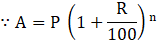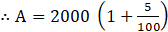2

A = 2000 (1+0.05)2

A = 2000 (1.05)2

A = 2000 (1.1025)

∴A= 2205/-

∴ C.I = A – P

∴ C.I = 2205 – 2000

C.I. = 205/-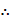Amount is 2205/- and Compound interest is 205/- .

b. Principal = 5000/-, Rate = 8% (p.c.p.a), Duration (n) = 3 years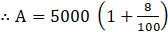3

A = 5000 (1 + 0.08)3

A = 5000 (1.08)3

A = 5000 (1.259712)

∴A= 6298.56/-

∵ C.I. = A - P

∴ C.I. = 6298.56 - 5000

C.I. = 1298.56/-Amount is 6298.56/- and Compound interest is 1298.56/- .

c. Principal = 4000/-, Rate = 7.5% (p.c.p.a), Duration (n) = 2 yearsA = 4000 (1 + 0.075)2

A = 4000 (1.075)2

A = 4000 (1.155625)

∴A= 4622.5/-

∵ C.I. = A - P

∴ C.I. = 4622.5 - 4000

C.I. = 622.5/-Amount is 4622.5/- and Compound interest is 622.5/- .

Question 2.

Sameerrao has taken a loan of ₹ 12500 at a rate of 12 p.c.p.a. for 3 years. If the interest is compounded annually then how many rupees should he pay to clear his loan?

Principal = 12500/-, Rate = 12% (p.c.p.a), Duration (n) = 3 years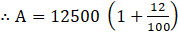3

A = 12500 (1+ 0.12)3

A = 12500 (1.12)3

A = 12500 (1.404928)A = 17561.60/-Sameerao has to pay an amount of 17561.60/- .

Principal = 8000/-, Rate = 10.5% (p.c.p.a), Duration (n) = 2 years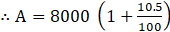2

A = 8000 (1+0.105)2

A = 8000 (1.105)2

A = 8000 (1.221025)

∴ A = 9768.2/-

∵ C.I. = A - P

∴ C.I. = 9768.2 - 8000

C.I. = 1768.2/-

∴ Shalaka has to pay a compound interest of 1768.2/- .

###### Practice Set 14.2
Question 1.

On the construction work of a flyover bridge there were 320 workers initially. The number of workers were increased by 25% every year. Find the number of workers after 2 years.

Present number of workers = 320 workers, Rate (increase) = 25% (p.c.p.a), Duration (n) = 2 years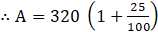2

A = 320 (1+0.25)2

A = 320 (1.25)2

A = 320 (1.5625)

∴A= 500/-

∴ The number of workers after 2 years will be 500.

Question 2.

A shepherd has 200 sheep with him. Find the number of sheeps with him after 3 years if the increase in number of sheeps is 8% every year.

Present number of sheeps (P) = 200 sheeps, Rate = 8% (p.c.p.a), Duration (n) = 3 years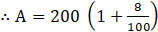3

A = 200 (1+0.08)3

A = 200 (1.08)3

A = 200 (1.259712)

∴A= 251.9424

A = 252 sheeps (Rounded off)

∴ The number of sheeps after 3 years is 252.

Question 3.

In a forest there are 40,000 trees. Find the expected number of trees after 3 years if the objective is to increase the number at the rate 5% per year.

Present Trees (P) = 40000 trees, Rate = 5% (p.c.p.a), Duration (n) = 3 years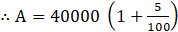3

A = 40000 (1+0.05)3

A = 40000 (1.05)3

A = 40000 (1.157625)

∴A= 46305/-

∴ The expected number of trees after 3 years will be 46305.

Question 4.

The cost price of a machine is 2,50,000. If the rate of depreciation is 10% per year find the depreciation in price of the machine after two years.

Principal = 250000/-, Rate (decrement) = 10% (p.c.p.a), Duration (n) = 2 years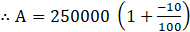2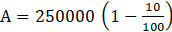2

A = 250000 (1-0.1)2

A = 250000 (0.9)2

A = 250000 (0.81)

∴A= 202500/-

∵ C.I. = A - P

∴ Depreciation in Price (C.I.) = 202500 – 250000

Depreciation in Price (C.I.) = -47500/-

(-) sign denotes the depreciation in amount.

∴ Depreciation in Price of the machine after 2 years will be 47500/- .

Question 5.

Find the compound interest if the amount of a certain principal after two years is
₹ 4036.80 at the rate of 16 p.c.p.a.

Amount= 4036.80/-, Rate = 16% (p.c.p.a), Duration (n) = 2 years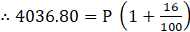2

4036.80 = P (1+0.16)2

4036.80 = P (1.16)2

4036.80 = P (1.3456)

∴ P = 3000/-

∵ C.I. = A - P

∴ C.I. = 4036.80 - 3000

C.I. = 1036.80/-Compound interest is 1036.80/- .

Question 6.

A loan of ₹ 15000 was taken on compound interest. If the rate of compound interest is 12 p.c.p.a. find the amount to settle the loan after 3 years.

Principal = 15000/-, Rate = 12% (p.c.p.a), Duration (n) = 3 years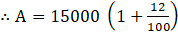3

A = 15000 (1+0.12)3

A = 15000 (1.12)3

A = 15000 (1.404928)

∴A= 21073.92/-Amount to settle the loan after 3 years is 21073.92/- .

Question 7.

A principal amounts to ₹ 13924 in 2 years by compound interest at 18 p.c.p.a. Find the principal.

Amount= 13924/-, Rate = 18% (p.c.p.a), Duration (n) = 2 years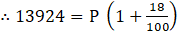2

13924 = P (1+0.18)2

13924 = P (1.18)2

13924 = P (1.3924)

∴ A = 10000/-

∴ The principal is 10000/- .

Question 8.

The population of a suburb is 16000. Find the rate of increase in the population if the population after two years is 17640.

Present Population (P) = 16000/-, Rate = R% (p.c.p.a), Duration (n) = 2 years

Population after 2 years (A) =17640/-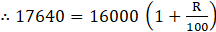2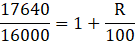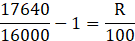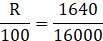∴R= 5%

∴ The population of that suburb will increase at the rate of 5% .

Question 9.

In how many years ₹ 700 will amount to ₹ 847 at a compound interest rate of 10 p.c.p.a.

Principal = 700/-, Rate = 10% (p.c.p.a), Duration (n) = n years Amount = 847/-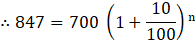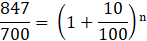1.21 =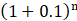1.21 =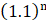∴ n = 2 years

∴ The number of years required to gain an amount of 847/- from a principal of 700/- is 2 .

Question 10.

Find the difference between simple interest and compound interest on ₹ 20000 at 8 p.c.p.a.

Principal = 20000/-, Rate = 8% (p.c.p.a), Duration (n) = n years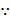For the first year, compound interest and simple interest will be same, so it will vary from second year, therefore assuming the duration as 2 years in the same case.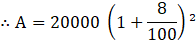A = 20000 (1+0.08)2

A = 20000 (1.08)2

A = 20000 (1.1664)

∴ A = 23328/-

∵ C.I. = A - P

C.I. = 23328 – 20000C.I. = 3328/-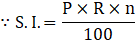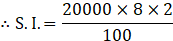S.I. = 3200/-

∴ Difference = C.I. – S.I.

Difference = 3328 – 3200

Difference = 128 /-

∴ The difference between simple interest and compound interest is 128/- .

## PDF FILE TO YOUR EMAIL IMMEDIATELY PURCHASE NOTES & PAPER SOLUTION. @ Rs. 50/- each (GST extra)

SUBJECTS

HINDI ENTIRE PAPER SOLUTION

MARATHI PAPER SOLUTION
SSC MATHS I PAPER SOLUTION
SSC MATHS II PAPER SOLUTION
SSC SCIENCE I PAPER SOLUTION
SSC SCIENCE II PAPER SOLUTION
SSC ENGLISH PAPER SOLUTION
SSC & HSC ENGLISH WRITING SKILL
HSC ACCOUNTS NOTES
HSC OCM NOTES
HSC ECONOMICS NOTES
HSC SECRETARIAL PRACTICE NOTES

2019 Board Paper Solution

HSC ENGLISH SET A 2019 21st February, 2019

HSC ENGLISH SET B 2019 21st February, 2019

HSC ENGLISH SET C 2019 21st February, 2019

HSC ENGLISH SET D 2019 21st February, 2019

SECRETARIAL PRACTICE (S.P) 2019 25th February, 2019

HSC XII PHYSICS 2019 25th February, 2019

CHEMISTRY XII HSC SOLUTION 27th, February, 2019

OCM PAPER SOLUTION 2019 27th, February, 2019

HSC MATHS PAPER SOLUTION COMMERCE, 2nd March, 2019

HSC MATHS PAPER SOLUTION SCIENCE 2nd, March, 2019

SSC ENGLISH STD 10 5TH MARCH, 2019.

HSC XII ACCOUNTS 2019 6th March, 2019

HSC XII BIOLOGY 2019 6TH March, 2019

HSC XII ECONOMICS 9Th March 2019

SSC Maths I March 2019 Solution 10th Standard11th, March, 2019

SSC MATHS II MARCH 2019 SOLUTION 10TH STD.13th March, 2019

SSC SCIENCE I MARCH 2019 SOLUTION 10TH STD. 15th March, 2019.

SSC SCIENCE II MARCH 2019 SOLUTION 10TH STD. 18th March, 2019.

SSC SOCIAL SCIENCE I MARCH 2019 SOLUTION20th March, 2019

SSC SOCIAL SCIENCE II MARCH 2019 SOLUTION, 22nd March, 2019

XII CBSE - BOARD - MARCH - 2019 ENGLISH - QP + SOLUTIONS, 2nd March, 2019

HSC Maharashtra Board Papers 2020

(Std 12th English Medium)

HSC ECONOMICS MARCH 2020

HSC OCM MARCH 2020

HSC ACCOUNTS MARCH 2020

HSC S.P. MARCH 2020

HSC ENGLISH MARCH 2020

HSC HINDI MARCH 2020

HSC MARATHI MARCH 2020

HSC MATHS MARCH 2020

SSC Maharashtra Board Papers 2020

(Std 10th English Medium)

English MARCH 2020

HindI MARCH 2020

Hindi (Composite) MARCH 2020

Marathi MARCH 2020

Mathematics (Paper 1) MARCH 2020

Mathematics (Paper 2) MARCH 2020

Sanskrit MARCH 2020

Important-formula

THANKS